Rounding numbers

How to round numbers

Rounding numbers makes a number look simpler, but it also makes it so that the number is no longer exact. Why do we sometimes round numbers?

Imagine if you were trying to pay for your meal and you had a can of pop that costed $1.09, a slice of pizza that costed$2.96, and a salad that costed $3.12. When it comes time to pay and you want to quickly figure out around how much you'll need to take from your wallet, you can round numbers to, for example,$1 + $3 +$3, which gives you an approximate amount of $7. It makes calculations easier. Rounding also makes it easier to describe and understand a numerical figure. For example, if you made$625 at your part-time job each month, you could instead say "I make around \$600 a month". Rounding still gives others a good idea of the number you're trying to convey, while making it simpler to grasp.

Another good reason to use rounding is that sometimes, you simply don't have to be exact. If someone approached you on the streets asking you where the nearest restaurant was, instead of saying it's a 26 minute walk, you could tell them it's about half an hour (30 minutes) away.

So how do we round numbers? Let's try out some practice problems.

How to round to the nearest whole number

Question:

Round the following number to the nearest: i) ten ii) hundred iii) thousand

9706

Solution:

1. Ten

So how will we be rounding numbers to the nearest ten? We know that finding the tens means we'll be focusing on the last two digits in the number.

9706

The method to rounding numbers is to decide on which part of the number to keep, and then round the other part. One of the more common ways to round numbers is to see if you'll need to round up or round down. A rule of thumb is that if the number is less than 5, we'll round down. If it's 5 or greater, we'll round up. Since "6" is more than 5, we'll be rounding up numbers:

9710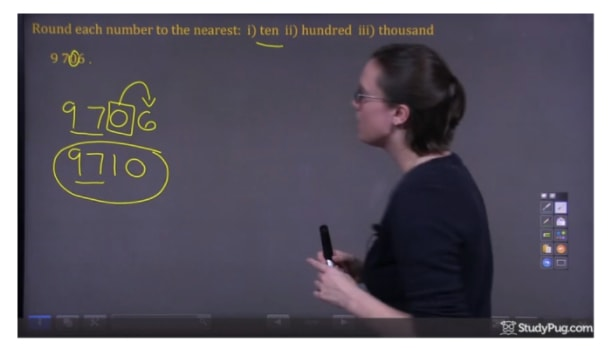Round the number to the nearest ten
1. Hundred

How about rounding numbers to the nearest hundred? As we're dealing with hundreds, focus on the second and third digit:

9706

Figure out which part of the number we'll want to keep, and then round the end.

9700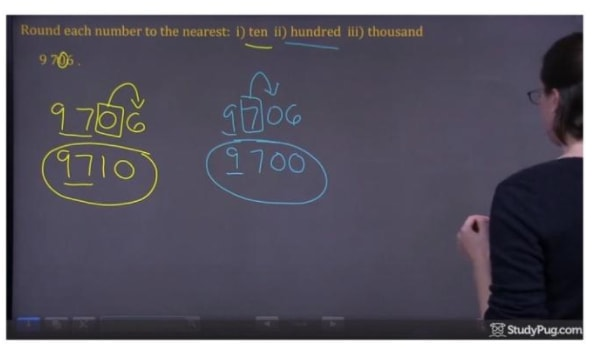Round the number to the nearest hundred
1. thousand

Now we focus on the first two digits,

9706

Rounding that number gives us:

10000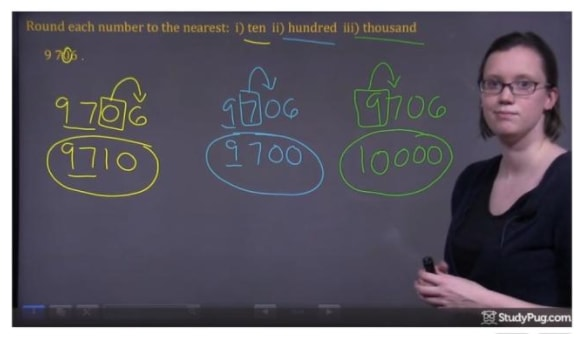Round the number to the nearest thousand

Now you've understood how rounding whole numbers work!

But what does rounding decimal numbers look like? Basically, it's the same concept as dealing with whole numbers. Let's take a look.

Question 2:

Round the number to the nearest i) tenth ii) hundredth iii) thousandth

0.4572

1. Tenth

Focus on the second and third digit,

0.4572

And then round the number

0.5000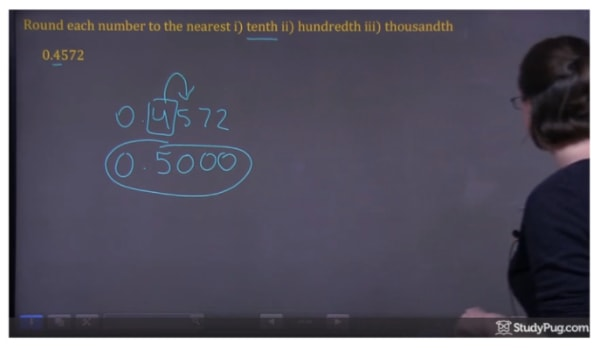Round the number to the nearest tenth
1. Hundredth

Focus on the third and fourth digit,

0.4572

And then round the number

0.4600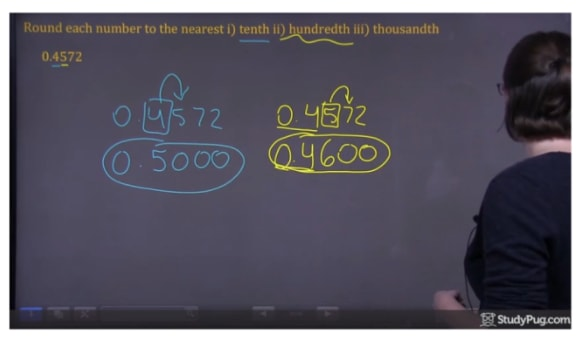Round the number to the nearest hundredth
1. Thousandth

Focus on the last two digits,

0.4572

And then round the number

0.4570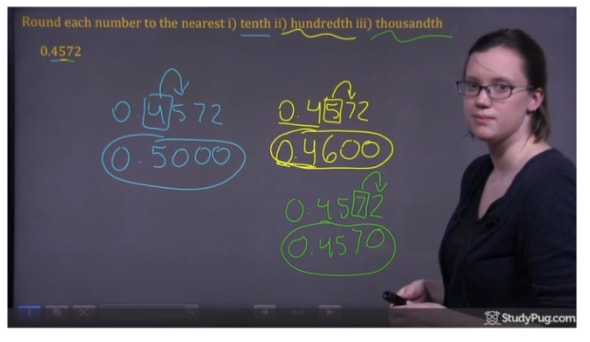Round the number to the nearest thousandth

Always remember to first identify which numbers you'll be considering when rounding (the place value). Then, use the rule of rounding up and down to determine what the final number will be.

Rounding numbers

In this section, we will be using our knowledge about the place value system to round both whole numbers and decimal numbers. Say your curfew is 10:00pm. You are running a bit late and you arrive home at 10:02pm, 2 minute past your curfew. You could round that time to 10:00pm. Phew, thank goodness for rounding! When rounding numbers, we first focus on the digit in the place we are asked to round. Those digits to the left of this place are left alone. The digit we are focusing on is also left alone if the next digit to the right is less than 5; however, the digit we are focusing on is increased by 1 if the next digit to the right is 5 or more.

Lessons

• Introduction
a)
What is Decimal Place Value?

• 1.
Round each number to the nearest: i) ten ii) hundred iii) thousand
a)
9 706

b)
33 782

• 2.
Round each number to the nearest i) tenth ii) hundredth iii) thousandth
a)
0.4572

b)
2.6308

c)
5.3962

• 3.
The table below shows the average populations of three islands in Hawaii as recorded between 2008 and 2010. Round each population to the nearest
 Island Population Oahu 653 207 Maui 144 444 Kauai 65 689
a)
100

b)
1000

c)
10 000

• 4.
Round each number to the nearest hundred
a)
Abby and her friend take a road trip from Toronto to Vancouver. The distance between these cities is approximately 4 382.042 km.

b)
The Challenger Deep, located in the Pacific Ocean, is the deepest part of the ocean. Challenger Deep is approximately 36 200 feet deep.

c)
Today in Science class, Carry learned that light travels at a speed of 299 792 458 m/s